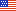An unofficial archive of your favorite United States government websiteOfficial websites do not use .rip
We are an unofficial archive, replace .rip by .gov in the URL to access the official website. Access our document index here.We are building a provable archive!
A lock () or https:// don't prove our archive is authentic, only that you securely accessed it. Note that we are working to fix that :)

# Computer Security Resource Center

A  |  B  |  C  |  D  |  E  |  F  |  G  |  H  |  I  |  J  |  K  |  L  |  M  |  N  |  O  |  P  |  Q  |  R  |  S  |  T  |  U  |  V  |  W  |  X  |  Y  |  Z

### security strength

Abbreviation(s) and Synonym(s):

Bits of security
Bits of Security
Security Strength

Definition(s):

A number associated with the amount of work (that is, the number of operations) that is required to break a cryptographic algorithm or system. In this policy, security strength is specified in bits and is a specific value from the set {80, 112, 128, 192, 256}.
Source(s):

A number associated with the amount of work (e.g., the number of operations) that is required to break a cryptographic algorithm or system.
Source(s):
NIST SP 800-102 under Security strength

See security strength.
Source(s):
NIST SP 800-107 Rev. 1 under Bits of security

A number associated with the amount of work (that is, the number of operations) that is required to break a cryptographic algorithm or system. If 2N execution operations of the algorithm (or system) are required to break the cryptographic algorithm, then the security strength is N bits.
Source(s):
NIST SP 800-107 Rev. 1 under Security strength

A measure of the computational complexity associated with recovering certain secret and/or security-critical information concerning a given cryptographic algorithm from known data (e.g. plaintext/ciphertext pairs for a given encryption algorithm). In this Recommendation, the security strength of a key derivation function is measured by the work required to distinguish the output of the KDF from a bit string selected uniformly at random from the set of all bit strings with the same length as the output of the KDF, under the assumption that the key derivation key is the only unknown input to the KDF.
Source(s):
NIST SP 800-108 under Security strength

A number associated with the amount of work (that is, the number of basic operations of some sort) required to break a cryptographic algorithm or system. Security strength is often expressed in bits. If the security strength is S bits, then it is expected that (roughly) 2S basic operations are required to break the algorithm or system.
Source(s):
NIST SP 800-133 Rev. 2 under Security strength
NIST SP 800-133 [Superseded] under Security strength
NIST SP 800-133 Rev.1 [Superseded] under Security strength

A number associated with the expected amount of work (that is, the base 2 logarithm of the number of operations) to cryptanalyze a cryptographic algorithm or system.
Source(s):
NIST SP 800-152 under Security strength

A number associated with the amount of work (that is, the number of operations) that is required to break a cryptographic algorithm or system.
Source(s):
NIST SP 800-56B Rev. 2 under Security strength
NIST SP 800-56A Rev. 2 [Superseded] under Security strength
NIST SP 800-56B Rev. 1 [Superseded] under Security strength

A number associated with the amount of work (that is, the number of operations of some sort) that is required to break a cryptographic algorithm or system in some way. In this Recommendation, the security strength is specified in bits and is a specific value from the set {112, 128, 192, 256}. If the security strength associated with an algorithm or system is S bits, then it is expected that (roughly) 2S basic operations are required to break it.
Source(s):
NIST SP 800-90A Rev. 1 under Security Strength

A number associated with the amount of work (i.e., the number of operations) that is required to break a cryptographic algorithm or system.
Source(s):
NIST SP 800-175B Rev. 1 under Security strength

A number associated with the amount of work (i.e., the number of operations) that is required to break a cryptographic algorithm or system. In this Recommendation, the security strength is specified in bits and is a specific value from the set {80, 112, 128, 192, 256}. Note that a security strength of 80 bits is no longer considered sufficiently secure.
Source(s):
NIST SP 800-57 Part 1 Rev. 5 under Security strength

A number associated with the amount of work (that is, the number of operations) that is required to break a cryptographic algorithm or system. In this Recommendation, the security strength is specified in bits and is a specific value from the set {80, 112, 128, 192, 256}
Source(s):
NIST SP 800-57 Part 1 Rev. 3 [Superseded] under Security strength

A number associated with the amount of work (that is, the number of operations) that is required to break a cryptographic algorithm or system. In this Recommendation, the security strength is specified in bits and is a specific value from the set {80, 112, 128, 192, 256}. Note that a security strength of 80 bits is no longer considered sufficiently secure.
Source(s):
NIST SP 800-57 Part 1 Rev. 4 [Superseded] under Security strength